In chemistry and biochemistry lab when you work with solutions you should know about how to make the solution? You should know about molecular mass, molar mass, molar volume, Molarity, and Molality and also how to make molar and Molal solutions? When you know about all these terms and their uses then you can make easily every type of solution either molar or Molal. Most of these lab techniques are studied in Analytical chemistry which is one of 5 branches of chemistry.

## What is Molarity?

The number of Moles of a substance dissolves per dm3 of the solution.

Symbol of Molarity: M

Formula:

Molarity = no of mass of solute/ vol of solution dm3

### Calculate the molarity of solution containing 20.7 g ok K2CO3 dissolved in 500 cm3 of the given solution?

Solution:

Mass of -0.7g

Molar mass =

Volume of solution= 500cm3= 0.5dm3  (500/1000= 0.5)

(For example, 1 dm3 = 1000 cm3. Another important rule is the definition 1 liter = 1 dm3. )

Molarity= 20.7g/138g/mol  1/ 0.5dm3

## Molar Solution

When one gram mole of solute dissolved per dm3 solution called molar solution.

## What is standard solution??

This is very important part of practical you should know about what is standard solution?

A solution of known molarity is called standard solution.

eg, 0.1 molar NaOH

0.05 molar HCl

Molality the number of moles of substance dissolves in one g of solutio

or

Number of moles of solute in one gram sololvent

Molarity = no of mass of solute/ vol of solution in kg

### What is molality of a solution prepared by dissolving 3g of toluene  in 250g benzene?

Solution

Mass of toluene = 3g

Mass of benzene = 250g = 0.250kg

Conversion in kg 250/1000= 0.250

Molar mass of toluene =

Molality= (mass of substance / mol mass of substance) 1/ vol of solution in kg

3g/92g/mol 1/0.25

Some important salts with their molecular formulas and wt.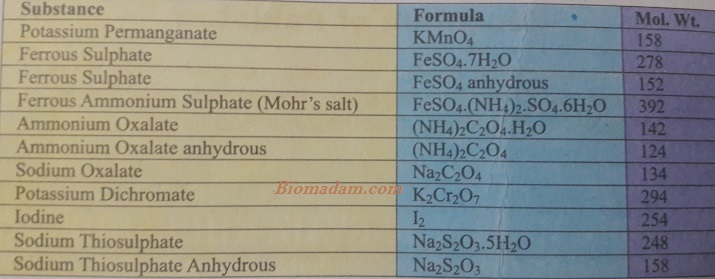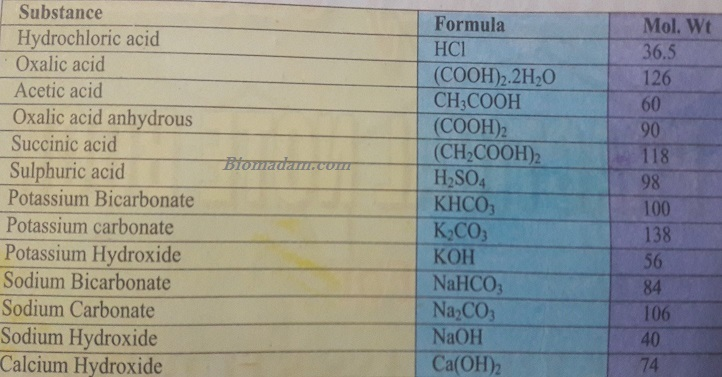Some important acids with concentrations.

Preparation of solutions according to following weights.

You can make 0.1 M solution of following salts by using given data.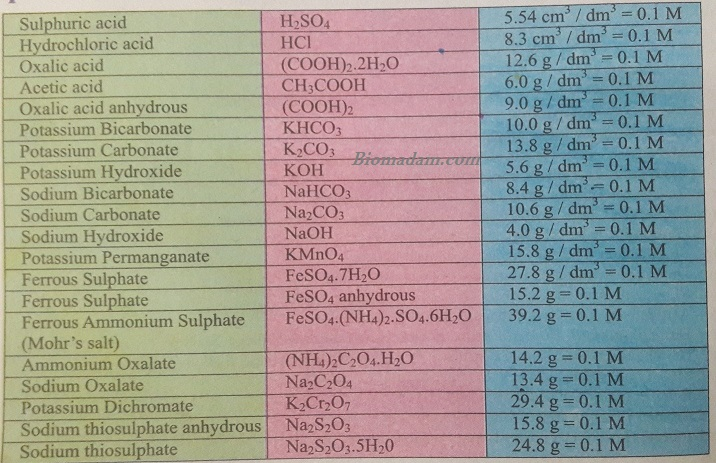When you know all about these things you can make easily each type of solution

### Following some solutions and Standard Curves are Prepared.

#### 1) How to prepare 100 ml solution of 1 molar glucose?

##### Apparatus:

Beaker, stirrer, weight balance, glucose, distilled water

##### Calculations:

Molar mass of glucose (C6H12O6) = 6(12) +12+6(16) =180 g

Amount of glucose required = [Molar mass / 1000] X volume required

Amount of glucose required = [180 / 1000] X 100 = 18 g

##### Procedure:
1. Weight 18g of glucose by using weight machine.
2. Take a beaker and add glucose in it.
• Add some amount of distilled water.
1. Dissolve it then make the volume up to 100ml.
2. Store in an appropriate container.

#### 2) How you can make 4% solution of glucose?

##### Principle:

Percentage composition is expressed as the solute and solvent amounts that are dissolved per 100 parts by the solution.

##### Apparatus:

Beaker, stirrer, electric weight machine, distilled water, glucose

##### Procedure:
1. Weight 4 g of glucose by using electric weight balance.
2. Add this calculated glucose in a beaker.
• Add 30-40 ml of distilled water to dissolve the glucose.
1. Stirrer the solution to dissolve the glucose.
2. Add more water to make the volume upto100ml.

Note: Gently heat the solution if the solute does not dissolve properly.

#### 3) Prepare phosphate buffer of pH 6.0, molarity 0.05, volume 100ml?

Buffer is a solution of weekly dissociated acid and its conjugated base, and vice versa.

##### Apparatus:

Beaker, weight balance, spatula, stirrer, KH2PO4, K2HPO4, distilled water, KOH or HCl solution, pH meter

Calculations:

pH= pKa + log[S]/ [A] ……  (1)

By putting the values in equation (1);

6.0 = 7.2 + log [S]/ [A]

6.0 – 7.2 = log [S]/ [A]

-1.2 = log [S]/ [A]

1.2 = log [A]/ [S]

Antilog 1.2 = log [A] / [S]

15.55 = [A] / [S]

15.55 / 1 = [A] / [S]

Number of moles of acid = [15.55 / 16.55] × 0.05= 0.046 M

Moles of salt = [1 / 16.55] × 0.05 = 0.003 M

Amount of required = [molecular mass/ 1000] × moles × volume required

Amount of acid required= [136 / 1000] × 0.046 × 100= 0.652g

Amount of salt = [174 / 1000] × 0.003 × 100 = 0.052g

##### Procedure:
1. Take a beaker and add weighed amount of acid and salt in it.
2. Add distilled water to make the volume approximately 90 ml and mix thoroughly.
• Check the pH of solution by pH meter.
1. Adjust the pH with corresponding acid or conjugate base if desired.
2. Make the volume up to 100 ml and store in appropriate container.

#### 4) How will you make Standard curve of glucose?

##### Procedure:
1. Weight 0.1 g glucose and dissolve it to make 100 ml.
2. Take 100, 200, 300, 400, 600 µL separately and make 10 ml with distilled water.
• Take 1 ml from this stock solution in separate test tube add 1 ml phosphate buffer of pH 6.8.
1. Add 3 ml DNS and boil it in water bath for 5 min.
2. Cool it to room temperature then measure its absorbance at 550 nm.
##### Calculations
 Sr. No Standard glucose solution (ul) H2O  (ml) Total volume   (ml)* Glucose concentration (ug/ml) Buffer (ml) DNS reagent (ml) Absorbance at 550 nm 1 100 9.9 10 10 1 3 0.03 2 200 9.8 10 20 1 3 0.10 3 300 8.7 10 30 1 3 0.31 4 400 9.6 10 40 1 3 0.75 5 600 9.4 10 50 1 3 1.2 Blank 000 10.0 10 00 1 3 00

*Take one ml from this solution and add 3 ml DNS

##### Standard curve graph Plot: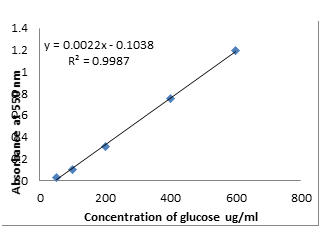Concentration of Glucose x= y+ 0.1038

0.0022

#### 5) Find out Standard curve of BSA for protein estimation?

##### Material:

Bovine serum albumin, Bradford reagent, distilled water

##### Equipment:

Spectrophotometer, rest tubes

##### Procedure:
• Take 0.1 g bovine serum albumin and dissolve it in distilled water to make 100 ml (1 mg/ml).
• Take 20, 40, 60, 80, 100 µl, separately and add water to make final volume 100 µl.
• Add one ml of Bradford reagent and mix to develop color.
• Take absorbance at 595 nm.
• Make a standard curve to quantify the protein content of unknown samples

Standard curve of bovine serum albumin for determination of total protein content.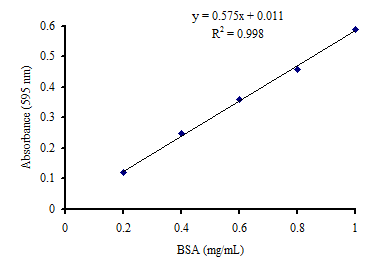After reading these calculations you will be able to understand and know some following questions.

## Questions?

### How much does 1 molecule of Glucose weigh?

C6H12O6180 g

180g/mol

Glucose

## How to make a Molar solution?

If you want to make molar solution of any substance you should know its quantity in which you want to make like 0.1 or o.01 molar and also know its molecular mass or molar mass

e.g, you want to make 0.1 molar solution of NaCl

NaCl has mol wt 58g/mol

=  5.8g/mol

Weight 5.8 g NaCl and dissolve in 1 liter water

This is 1 molar solution

Same method for other concentrations

This method apply only for solid solutionsHifza is a student of bioinformatics. She is a research student and working on cancer.

Pin It